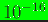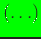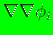## Linking external programs with the max-ent library

If one wants to use the max-ent library with any other program, then first create the static library file libmaxent.a by invoking make maxentlib in the top-directory. Let the environment variable MAXENTDIR point to where MAXENT-V1.4 is located. The library file is at $(MAXENTDIR)/MAXENT-V1.4/lib/libmaxent.a. To link this library file with any other Fortran 90 program, the following command can be used: g95 -o a.out <object-files> -L$(MAXENTDIR)/MAXENT-V1.4/lib -lmaxent

If an interface from a C or C ++ program is required, the appropriate call (depending on the compiler and the operating system) should be invoked. The subroutine call from a Fortran 90 program to compute the max-ent basis functions is given below (see main.f90 and also maxent.f90 for further details):

call drivermaxent(n,nsd,scheme,prior,xyz,p,rmax,D,maxit,eps,printflag,errorflag,lengthscale,dettol,phi,dphi,ddphi)
The input parameters in the above subroutine are as follows:
1. n : number of neighbors of point(a positive integer);
2. nsd : spatial dimension (a positive integer);
3. scheme : either descent, newton, or lbfgs (a string);
4. prior : either uniform, cubic, quartic, gaussian, or gaussian-rbf (a string);
5. xyz : nodal coordinates of theneighbors (double precision array xyz(n,nsd));
6. p : coordinates of the point(double precision array p(nsd));
7. rmax : support sizes of theneighbors (double precision array rmax(n));
8. D : anisotropic metric (double precision array D(nsd,nsd,n));
9. maxit : maximum number of iteration (a positive integer);
10. eps : convergence tolerance (double precision);
11. printflag : print convergence information (logical: .true. or .false.);
12. errorflag : error flag (pass,Hessian too small;non-convergence in maxit iterations);
13. lengthscale : length scale for domain (optional argument) so that convergence criterion is independent of the magnitude of nodal coordinates;
14. dettol : determinant tolerance (optional argument) with default ofThe output variables (phi, dphi, and ddphi) in the parameter-list are defined as optional:
1. phi : basis function vector (double precision phi(n));
2. dphi : 1st derivatives of basis functions (double precision dphi(nsd,n));
3. ddphi : 2nd derivatives of basis functions (double precision ddphi(nsd*nsd,n));
Please note that any calling subroutine must first determine for every point, the number of neighborsfor, assign the nodal coordinates of thesenodes to the array xyz, and the support sizes of thesenodes must be assigned to the array rmax. Then only can a call to the subroutine drivermaxentbe invoked. It is assumed that computations for dphi and/or ddphi are requested in conjunction with phi. Hence, in the parameter-list, phi (is computed), phi and dphi (andare computed), phi and ddphi (andare computed), or all three (,andare computed) are valid.

N. Sukumar